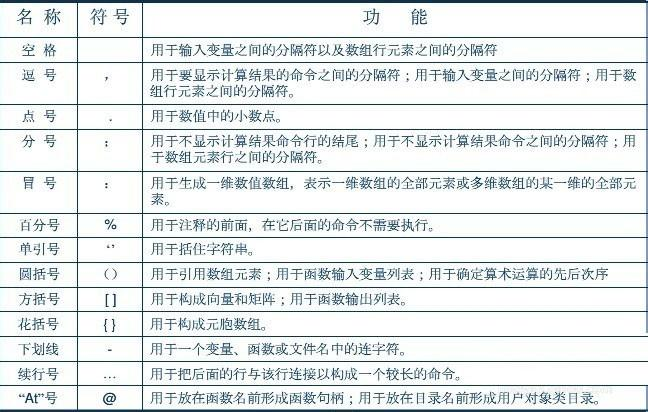• ## Matlab分号的使用

千次阅读 2018-09-24 19:56:27
运行matlab程序的时候，程序中变量的值会自动储存在 workspace中。 程序命令后面输入与不输入“;”的区别是，当输入半角的分号时，命令中的变量会自动列表储存在 workspace中，变量的值不会在window command中显示...
后面的加分号时，运行结果在命令窗口不显示。 不加分号时，当点击回车时，运行结果就会立即显示出来！ 运行matlab程序的时候，程序中变量的值会自动储存在 workspace中。

程序命令后面输入与不输入“;”的区别是，当输入半角的分号时，命令中的变量会自动列表储存在 workspace中，变量的值不会在window command中显示出来；当不输入半角的分号时，变量不仅也会自动储存在workspace中，还会在window command窗口中显示出变量的值。


展开全文• matlab分号、冒号、逗号等常用标点符号的作用，供学习参考 其中冒号的常用法总结 ： 1）a:b 表示[a,a+1,……,b] >> A=1:6 A =  1 2 3 4 5 6 2）当然如果b和a不是整数的话，则向量的最后一...
matlab中分号、冒号、逗号等常用标点符号的作用，供学习参考

其中冒号的常用法总结 ：

1）a:b   表示[a,a+1,……,b]

>> A=1:6

A =

1     2     3     4    5    6

2）当然如果b和a不是整数的话，则向量的最后一位数是n+a，且n=fix（b-a）

>> A=1.2:5.2

A =

1.2000    2.2000    3.2000    4.2000    5.2000

3）上面的前提是a<b，如果a>b则会出现空值

>> A=6:1

A =

Empty matrix: 1-by-0

此时 A是空矩阵，从workspace也可以看到A

4）a:c:b表示[a,a+c,……,a+n*c],其中n=fix((b-a)/c)

>> A=1:3:11

A =

1     4     7    10

5)A(:)表示以一列的方式显示A中所有元素

>> A=[1 2 3;4 5 6]

A =

1     2     3

4     5     6

>> A(:)

ans =

1

4

2

5

3

6

6）b=A(i, :)表示把矩阵A的第i行存入b

>> b=A(1,:)

b =

1     2     3

7）b=A( :,j)表示把矩阵A的第j列存入b

>> b=A(:,1)

b =

1

4

8）b=A(J :K)表示把矩阵A中[A(J),A(J+1),...,A(K)]这些元素存入b中

>> b=A(3:5)

b =

2     5     3

9）b=A（:,c:d）表示把矩阵A的第c到第d列存入b中，当然c，d大于A的列数则出错（b=A（c:d,:）表示取行）

>> A=[1 2 3;4 5 6;7 8 9]

A =

1     2     3

4     5     6

7     8     9

>> b=A(:,2:3)

b =

2     3

5     6

8     9


展开全文冒号
• Matlab中,冒号“:”和分号“;”等常用标点符号分别有什么功能?...matlab分号、冒号、逗号等常用标点符号的作用如图，供学习参考！其中冒号的常用法总结 ：1)a:b 表示[a,a+1,……,b]>> A=1:6A =1 2 ...
在Matlab中,冒号“:”和分号“;”等常用标点符号分别有什么功能?应该怎么使用？接下来小编就给大家分享matlab中分号、冒号、逗号等常用标点符号的功能和用法总结，教你正确使用Matlab中的标点符号。matlab中分号、冒号、逗号等常用标点符号的作用如图，供学习参考！其中冒号的常用法总结 ：1)a:b   表示[a,a+1,……,b]>> A=1:6A =1     2     3     4    5    62)当然如果b和a不是整数的话，则向量的最后一位数是n+a，且n=fix(b-a)>> A=1.2:5.2A =1.2000    2.2000    3.2000    4.2000    5.20003)上面的前提是ab则会出现空值>> A=6:1A =Empty matrix: 1-by-0此时 A是空矩阵，从workspace也可以看到A4)a:c:b表示[a,a+c,……,a+n*c],其中n=fix((b-a)/c)>> A=1:3:11A =1     4     7    105)A(:)表示以一列的方式显示A中所有元素>> A=[1 2 3;4 5 6]A =1     2     34     5     6>> A(:)ans =1425366)b=A(i, :)表示把矩阵A的第i行存入b>> b=A(1,:)b =1     2     37)b=A( :,j)表示把矩阵A的第j列存入b>> b=A(:,1)b =148)b=A(J :K)表示把矩阵A中[A(J),A(J+1),...,A(K)]这些元素存入b中>> b=A(3:5)b =2     5     39)b=A(:,c:d)表示把矩阵A的第c到第d列存入b中，当然c，d大于A的列数则出错(b=A(c:d,:)表示取行)>> A=[1 2 3;4 5 6;7 8 9]A =1     2     34     5     67     8     9>> b=A(:,2:3)b =2     35     68     9教程结束，以上就是关于matlab中分号、冒号、逗号等常用标点符号的功能和用法总结，希望大家看完有所收获!更多matlab相关教程，请继续关注我们网站！
展开全文• matlab分号、冒号、逗号等常用标点符号的作用如图，供学习参考 其中冒号的常用法总结 ： 1）a:b 表示[a,a+1,……,b] >> A=1:6 A =  1 2 3 4 5 6 2）当然如果b和a不是整数的话，则向量...

matlab中分号、冒号、逗号等常用标点符号的作用如图，供学习参考其中冒号的常用法总结 ：

1）a:b   表示[a,a+1,……,b]

>> A=1:6

A =

1     2     3     4    5    6

2）当然如果b和a不是整数的话，则向量的最后一位数是n+a，且n=fix（b-a）

>> A=1.2:5.2

A =

1.2000    2.2000    3.2000    4.2000    5.2000

3）上面的前提是a<b，如果a>b则会出现空值

>> A=6:1

A =

Empty matrix: 1-by-0

此时 A是空矩阵，从workspace也可以看到A

4）a:c:b表示[a,a+c,……,a+n*c],其中n=fix((b-a)/c)

>> A=1:3:11

A =

1     4     7    10

5)A(:)表示以一列的方式显示A中所有元素

>> A=[1 2 3;4 5 6]

A =

1     2     3

4     5     6

>> A(:)

ans =

1

4

2

5

3

6

6）b=A(i, :)表示把矩阵A的第i行存入b

>> b=A(1,:)

b =

1     2     3

7）b=A( :,j)表示把矩阵A的第j列存入b

>> b=A(:,1)

b =

1

4

8）b=A(J :K)表示把矩阵A中[A(J),A(J+1),...,A(K)]这些元素存入b中

>> b=A(3:5)

b =

2     5     3

9）b=A（:,c:d）表示把矩阵A的第c到第d列存入b中，当然c，d大于A的列数则出错（b=A（c:d,:）表示取行）

>> A=[1 2 3;4 5 6;7 8 9]

A =

1     2     3

4     5     6

7     8     9

>> b=A(:,2:3)

b =

2     3

5     6

8     9

展开全文• 分号MATLAB和python中的区别 分号MATLAB中表示语句结果不显示，在python中用于分隔语句，如果要在一行中书写多条句，就必须使用分号分隔每个语句，否则Python无法识别语句之间的间隔。
• $(".cart").droppable({ drop: function () {$(this)//这里为什么不能加分号？？？？ .addClass("focus")//这里为什么不能加分号？？？？ .find("#tip").html(""); } }) }); 搞不清楚什么时候该加什么时候不该...
• 在运行matlab程序代码时，程序中的变量的值都会自动存储在workspace中。 而分号是否加的区别在于：代码后加分号，运行结果不会显示在命令窗口； 代码后不加分号，当点击回车时，运行结果会立即在命令窗口（window ...
• 刚接触 matlab的新手一定会有这样的疑问，为什么有些语句是以分号（;）结束，而有些却是逗号（ , ）呢？ 先放张百度经验的图 ↓ 在matlab中，如果语句的结尾是逗号，则会把赋值语句输出到命令窗口；分号不会。 ...
• 运行matlab程序的时候，程序中变量的值会自动储存在 workspace中。程序命令后面输入与不输入“;”的区别是，当输入半角的分号时，命令中的变量会自动列表储存在 workspace中，变量的值不会在window command中显示...
• 今天小鱼分享的比较简单，但是自己老搞混，所以记录下来，方便...看到这里就应该可以知道，matlab中行和列的分开标志是找逗号，有逗号就是行列分开，但如果没有逗号是什么意思呐，看下面 已行的形式表示并取第i行到
• 在代码行的后加分号，运行结果不会显示在命令行窗口； 不加分号，变量的值会随着运行显示在命令行窗口。 如果不想命令行窗口显示太多东西，可以在代码编写的过程中加上分号，需要知道变量的值的时候在工作区进行...
• 使用中括号[]表示数组时，分号;用来隔开行。用法如图。 使用中括号[]还可以拼接数组。如下，我们有两个数组A和B. [A,B]相当于水平拼接A和B，即horzcat(A,B) [A;B]相当于垂直拼接A和B，即vertcat(A,B) 具体见以下...
• a=[1:5]' %冒号 b=cumsum（[1:a]）%冒号 产生一个值 b=cumsum（[1;a]） %分号 产生6×1个值 注意区分matalb中的冒号和分号，看起来很相似~
• 刚才用matlab进行仿真，遇到一个十分糟心的问题，我所有代码都加了分号，但是运行的时候，命令行窗口还是在不断打印数据，重启matlab，重启电脑都试过了，还是不行。偏偏输出的数据还十分多，体验糟透了，百度也没有...bug
• 在 'linevals' 中返回一个结构，其中包含行号和可能缺少终端分号的候选行的冒犯字符串。 以通常不会在命令行产生输出的关键字（例如，'if'、'return'、'continue' 等）开头的行将被跳过。 TARGETFILES：输入可以是...
• 使用此函数读取以分号作为分隔符的 .csv 文件，并使用“,”而不是“.”写入值。 作为分隔符，还可以连接不同文件中不同变量的值。 在此更新版本中，参考选择得到纠正。 此函数将读取选定的“循环”文件，然后获取...
• 3.分号 （1）用于指令后，不显示计算结果 A=[1 2 3];B=[0 0 1] B = 0 0 1 （2)用于矩阵，作为行与行之间的分隔符 A=[6 5 4;7 8 9] A = 6 5 4 7 8 9 4.百分号用于注释 5.大括号  ...
• ## Matlab

2020-09-26 10:26:43
分号分割行 逗号分割列 &冒号循环生成一维矩阵 b = 1:1:10 ; 循环十次 部长为1 一维矩阵 &使用函数生成 linspace(开始，结束，元素个数)，等差生成指定元素的一维矩阵，省略个数则生成100个 c
• 此函数根据配置文件数据查找产生非抑制输出（不以分号终止）的代码行。 只考虑实际调用的函数。 使用： 简介; YourFunct(a,b,c); 配置文件关闭； p=profile('信息'); findNonSuppressedOutput( p, '/code/...
• ## MATLAB教程（1） MATLAB 基础知识

万次阅读 多人点赞 2017-10-26 20:57:32
去年看过一点点MATLAB，很久不用，遗忘惊人。为了加深自己的印象，扎实基础，现将官网上的基础教程做简单的翻译。 首先，以下从九个部分简单介绍基础入门知识。第一部分：MATLAB显示桌面的基本布局...
• ## MATLAB

2020-08-13 21:18:13
clc——两条一起使用，起到初始化的作用，防止之前的结果对新脚本文件产生干扰。 输出函数：disp()函数，不论加不加分号其结果都输出 按上下键调出之前使用过的代码 MATLAB中字符串的合并：strcat(“字符串1”...
•入门
• ## matlab

千次阅读 2015-01-23 09:22:27
x=linspace(0,2*pi)在matlab中是什么意思 从0到2pi按线性分50个点，50是默认 http://anony3721.blog.163.com/blog/static/51197420111013105344894/ http://blog.sina.com.cn/s/blog_4c8287230100ol0p.html Matlab......

# matlab分号的作用matlab 订阅